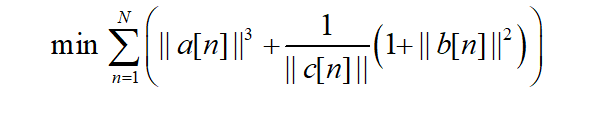# Cannot perform the operation: {convex} .* {convex}

Hi,
My objective function isand the constraints are just linear inequality constraints. It’ a convex problem.
I am having quite some trouble to implement this function. The error I get is “Cannot perform the operation {convex}{convex}”. I wrote it as below,
for n=1:N
E(1,n)=pow_pos(norm(a(:,n)),3) + inv_pos(c(1,n))
(1+pow_pos(norm(b(:,n)),2));
end
minimize sum(E)

Any suggestions on how to improve the code are welcome as well.

Thanks

2 Likes

Use `quad_over_lin` for ||b(n)||^2/max(c,0) if that indeed matches what you want.

1 Like

where b is a matrix of 2 rows and N columns and c is a vector of 1 row and N columns
But there is still an error：The first argument must be affine.

`quad_over_lin(b(:,n),c(n))`

quad_over_lin Sum of squares over linear.
Z=quad_over_lin(X,Y), where X is a vector and Y is a scalar, is equal to
SUM(ABS(X).^2)./Y if Y is positive, and +Inf otherwise. Y must be real.

``````If X is a matrix, quad_over_lin(X,Y) is a row vector containing the values
of quad_over_lin applied to each column. If X is an N-D array, the operation
is applied to the first non-singleton dimension of X.

quad_over_lin(X,Y,DIM) takes the sum along the dimension DIM of X.
A special value of DIM == 0 is accepted here, which is automatically
replaced with DIM == NDIMS(X) + 1. This has the effect of eliminating
the sum; thus quad_over_lin( X, Y, NDIMS(X) + 1 ) = ABS( X ).^2 ./ Y.

In all cases, Y must be compatible in the same sense as ./ with the squared
sum; that is, Y must be a scalar or the same size as SUM(ABS(X).^2,DIM).

Disciplined convex programming information:
quad_over_lin is convex, nonmontonic in X, and nonincreasing in Y.
Thus when used with CVX expressions, X must be convex (or affine)
and Y must be concave (or affine).``````

Hi, tammy. When i used quad_over_lin(norm(A(m,n)),b(m,n)), I encountered the same error：The first argument must be affine, which was the same as your. Could you please tell me how you finally solved this problem?

1 Like

Hello, I meet the same question as you proposed before. Could you please post the solutions for this question?

@blythe If you want help help, you need to show us the problem you are trying to solve. The first thing you must do, though, is to prove that your problem is convex.

thank u so much,i have solved the problem by using quad_over_lin

Hi, tammy,I encountered the same error： [Cannot perform the operation: {convex} .* {convex}],but I can’t deal with the coefficient 1 added to the front, can I see the form of your equation in matlab?